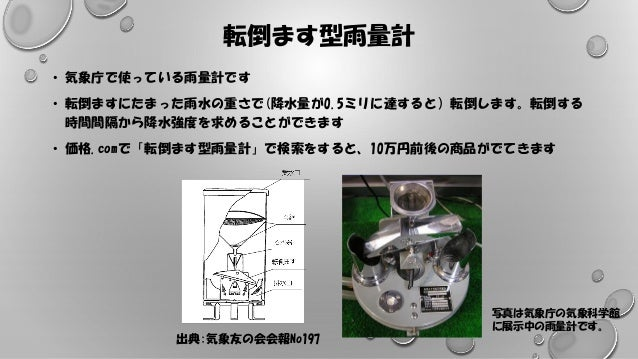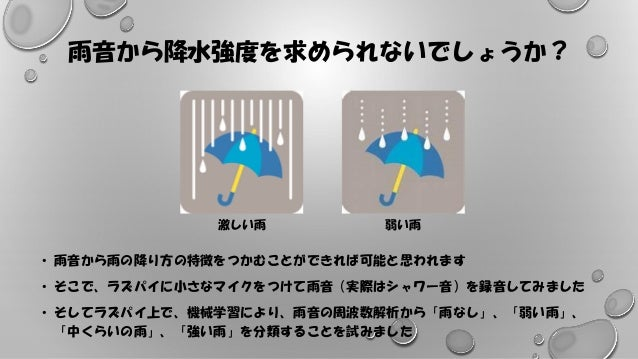Successfully reported this slideshow.Upcoming SlideShare
×

ofUpcoming SlideShare
Next

0 Likes

Share

# 2020年1月25日 AITCシニア成果発表会(5) 自分専用アメダス

＜＜AITCシニア技術者勉強会＞＞
チーム名：自分専用アメダス

See all

### Related Audiobooks

#### Free with a 30 day trial from Scribd

See all
• Be the first to like this

### 2020年1月25日 AITCシニア成果発表会(5) 自分専用アメダス

1. 1. 音による降水強度測定の試み 自分専用のアメダスを作るために･･･ 2020年1月25日 AITC勉強会合同発表会 チーム名：自分専用のアメダス 大和田浩美
2. 2. 転倒ます型雨量計 • 気象庁で使っている雨量計です • 転倒ますにたまった雨水の重さで(降水量が0.5ミリに達すると）転倒します。転倒する 時間間隔から降水強度を求めることができます • 価格.comで「転倒ます型雨量計」で検索をすると、10万円前後の商品がでてきます 写真は気象庁の気象科学館 に展示中の雨量計です。 出典:気象友の会会報No197
3. 3. 雨音から降水強度を求められないでしょうか？ • 雨音から雨の降り方の特徴をつかむことができれば可能と思われます • そこで、ラズパイに小さなマイクをつけて雨音（実際はシャワー音）を録音してみました • そしてラズパイ上で、機械学習により、雨音の周波数解析から「雨なし」、「弱い雨」、 「中くらいの雨」、「強い雨」を分類することを試みました 激しい雨 弱い雨
4. 4. ラズパイを使った雨量計！ 簡単でごめんなさい ラズパイ USBマイク (税込399円) モバイル バッテリー 100円ショップ で購入した入れ 物に収めました ふたの上にシャ ワーをかけて音 を録音します
5. 5. 音を採取しました 弱い雨 中くらいの雨 強い雨 弱 中 強 #!/bin/sh date=`date “+%Y%m%d%H%M”` amixer sset Mic 62 –c 1 arecord –D plughw:1.0 –f cd –r 16000 rec\${date}.wav サンプリング周波数: 16000Hz
6. 6. 周波数解析の結果 周波数ごとに振幅スペクトルを平均し たグラフ。サンプル数は、 雨なし: 271 弱い雨: 310 中くらいの雨:295 強い雨: 296 グラフでは、それぞれの特徴がでてい るようです 参考: Pythonで科学計算ライブラリ「numpy」を用いて周波数解析 (https://tomosoft.jp/design/?p=11527)
7. 7. 機械学習での判別をしてみました 入力データ ALM列はyで、 雨なし: 0 弱い雨: 1 中くらいの雨:2 強い雨: 3 A～ALL列はxで、各周波数の振幅スペクトル
8. 8. 機械学習での判別をしてみました Pythonのコード import pandas as pd #pandasをインポート import numpy as np #numpyをインポート from sklearn import model_selection #データ分割のためのmodel_selectionをインポート from keras.models import Sequential #keras.modulesからSequentialをインポート from keras.layers import Dense, Activation #keras.layersからDence,Activationをインポート rain_data = pd.read_csv(“rain_total.csv”,sep=“,”) #全データ読み込み X = rain_data.drop([‘class’],axis=1) #Xとして各周波数ごとの振幅スペクトルを Y = rain_data[‘class’] #Yとして降水強度のクラスを指定する data_train, data_test, label_train, label_test = model_selection.train_test_split(X,Y) #全データをトレーニングデータ(70%)とテストデータ(30%)に分ける #7:3の分割はデフォルト。シャッフルしてから分けてくださる print(len(data_train),len(data_test)) #トレーニングデータの個数を確認 print(len(label_train),len(label_test)) #テストデータの個数を確認
9. 9. 機械学習での判別をしてみました Pythonのコード（続き） model = Sequential() #シーケンシャルモデルを定義 model.add(Dense(500, input_dim=1000)) #中間層の数500、入力層の数1000 model.add(Activation(‘sigmoid’)) #sigmoidを活性化関数に model.add(Dense(4, input_dim=500)) #出力層の数4 model.add(Activation(‘softmax’)) #softmaxを活性化関数に model.compile(optimizer='adam',loss='sparse_categorical_crossentropy',metrics=['accuracy’]) #optimizerにadamを指定など model.fit(data_train, label_train, nb_epoch=20, batch_size=10) #epoch数20、batch size10で学習 model.metrics_names res = model.evaluate(data_test, label_test, batch_size=1) #テストデータで評価 print(res) #評価結果の確認 print(model.predict(data_test)) #テストデータのXでy’を予測 pre = np.argmax(model.predict(data_test),axis=1) #出力層で一番大きな値をy’とする print(pre) #y’を確認 print(label_test) #テストデータのyを確認 count = 0 for index in range(len(pre)): #各テストデータのｙとy’が同じ値なら1として if pre[index] == label_test.values[index]: #正解数をカウントする count = count + 1 print(count,‘/’,len(pre)) #「正解数/全テストデータ数」を確認
10. 10. 機械学習での判別をしてみました 結果 テストデータの loss テストデータ のaccuracy 正解数/テストデータ数 1回目 0.0965 0.959 281/293 2回目 0.1460 0.939 275/293 3回目 0.1636 0.952 279/293 4回目 0.1176 0.956 280/293 5回目 0.1308 0.956 280/293 テストデータ数は同じですが、その都度シャッフルしているため、そ れぞれのトレーニングデータ、テストデータは異なります。
11. 11. まとめ • 雨音から降水強度を求める最初の試みとして以下のことを試してみました • USBマイクをつけたラズパイで雨音を録音 • 録音したwavファイルから周波数解析。雨なし、弱い雨、中くらいの雨、強い雨に ついてデータを作成 • 上記データをトレーニングデータとテストデータに分けて機械学習 • 94%以上の正答率が出たので、まったくおかしな試みではないと思いました • ラズパイ起動時に過去のデータセットで機械学習を行い、そのモデルを使ってリアルタ イムに降水強度を推定し、結果を可視化するシステムができれば、自分専用のアメダス も夢ではないかもしれません

Total views

467

On Slideshare

0

From embeds

0

Number of embeds

1

0

Shares

0